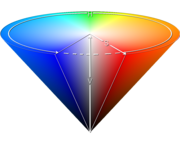## >Vision system

Introduction

Functions

Recording and replaying .arv video files

Introducing pYUV : a free player for YUV422 images and videos

Tutorial

Appendix

 << Previous

## What is the HSY color space?

Inspired by the HSV and HSL color spaces and optimized for speed on embedded systems.Why to code the Hue on 360° (9 bits) and so use a 16 bits word when you can code it on 256 values (8 bits)?

For computing saturation, we are using the HSV equation, faster to compute than the HSL one: S = (Max(R,G,B) - min(R,G,B)) / Max(R,G,B).

Finally, both Value and Lightness are not satisfying compared to a true luminance (they don’t use all three RGB components for their computation), so let’s use Y component since it is native from the camera.

 << Previous